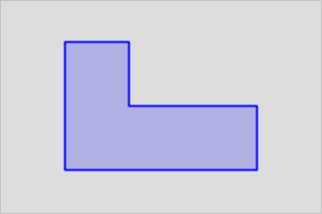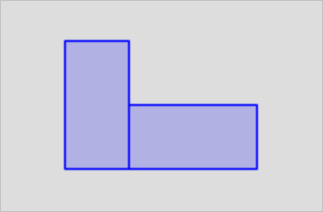This topic describes the ST_Union function. This function constructs a geometry object that represents the union of multiple specified geometry objects.

## Syntax

``````geometry  ST_Union(geometry set  g1Field);
geometry  ST_Union(geometry  g1 , geometry  g2);
geometry  ST_Union(geometry[]  g1Array);
geometry  ST_Union(geometry set  g1Field, float8 gridsize);
geometry  ST_Union(geometry set  g1Field, cstring options);
geometry  ST_Union(geometry set  g1Field, float8 gridsize, cstring options);
geometry  ST_Union(geometry[]  g1Array, float8 gridsize);
geometry  ST_Union(geometry[]  g1Array, cstring options);
geometry  ST_Union(geometry[]  g1Array, float8 gridsize, cstring options);``````

## Parameters

Parameter Description
g1field The fields of the geometry objects that you want to specify in the dataset.
g1 The first geometry object that you want to specify.
g2 The second geometry object that you want to specify.
g1Array An array that consists of the geometry objects.
gridsize The size of the grid that you want to specify. This parameter is used to merge vertices in the same grid when you perform a union operation. The default value is -1.0, which indicates that this parameter is disabled.
options The status of the parallel computing feature and the degree of parallelism that you want to specify. This parameter is used to enable the parallel computing feature and determine the degree of parallelism.

If you enable the feature, you must specify the value of this parameter as a JSON string, such as `'{"parallel": 4}'`. The degree of parallelism ranges from 1 to 64. The example value indicates that the parallel computing feature is enabled and the degree of parallelism is 4. The default value is `'{}'`, which indicates that the sequential computing feature is used.

## Description

• The output type of this function is MULTI or GeometryCollection. The ST_Union function has two versions:
• Version 1: The input of the ST_Union function is two geometry objects. The output of the ST_Union function is a MULTI, NON-MULTI, or GeometryCollection object. If one of the two geometry objects is NULL, the ST_Union function returns NULL.
• Version 2: The ST_Union function works as an aggregate function. The input is a collection of geometry objects. The output can be a MULTI or NON-MULTI object.
• The ST_Collect function and the ST_Union function are interchangeable. The ST_Union function tries to dissolve the boundaries of the specified geometry objects to check whether the constructed MultiPolygon object has intersecting parts. Therefore, in most cases, the ST_Union runs at a lower speed than the ST_Collect function.

## Examples

• The following example shows the differences between the ST_Union function and the ST_Collect function:
``````SELECT ST_Union(g1,g2),ST_Collect(g1,g2)
from (select 'POLYGON((0 0,1 0,1 2,0 2,0 0))'::geometry as g1,'POLYGON((1 0,3 0,3 1,1 1,1 0))'::geometry as g2) as t;``````• The following example shows the calculations with different configurations of the gridsize and options parameters:
``````-- Specify the gridsize parameter.
select st_area(st_union(geom, 0.005)) from tableA;

-- Specify the options parameter.
select st_area(st_union(geom, '{"parallel": 4}'::cstring)) from tableA;

-- Specify the gridsize and options parameters at a time.
select st_area(st_union(geom, 0.005, '{"parallel": 4}'::cstring)) from tableA;``````